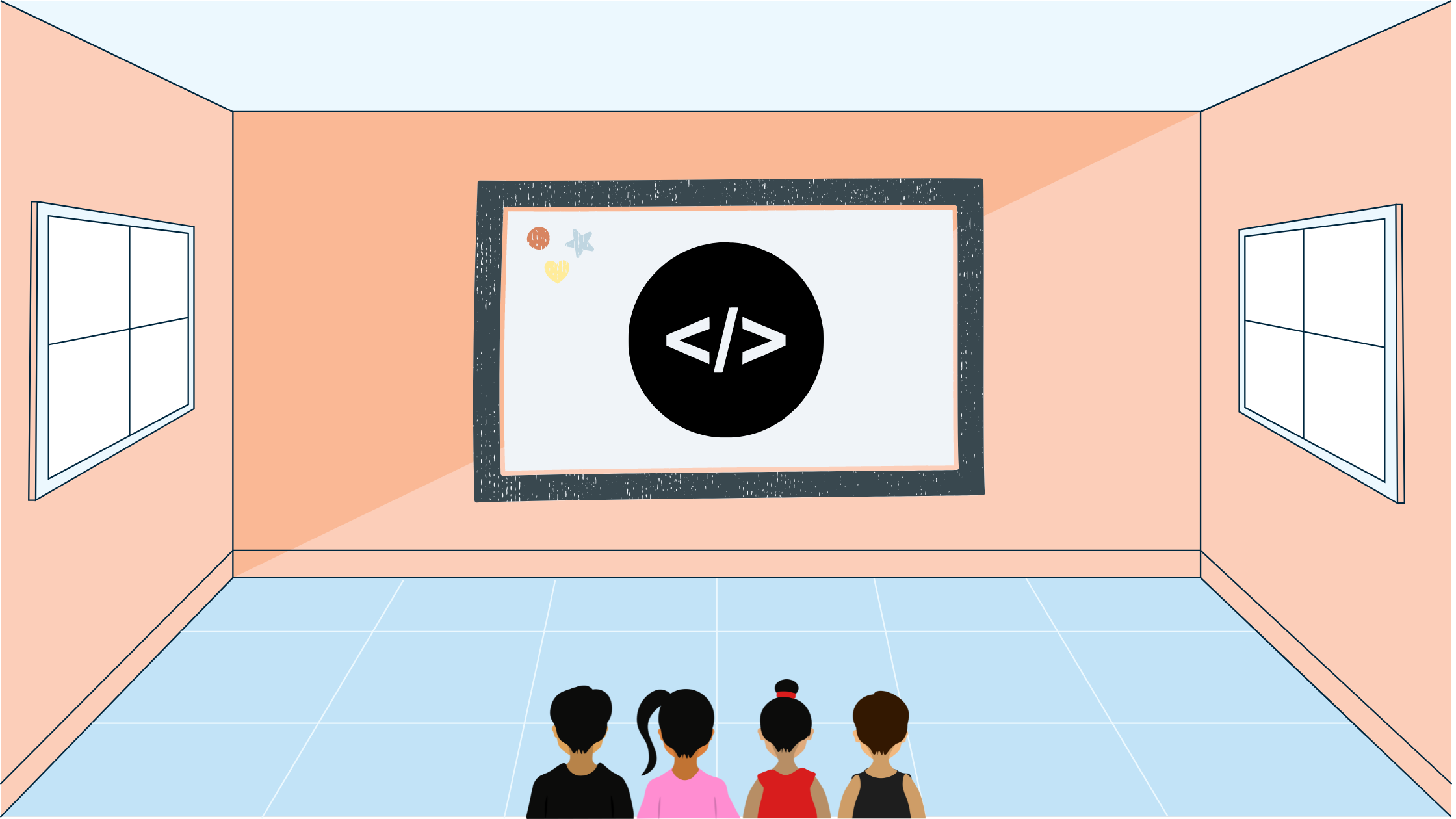Yash Singh# USACO Blocks

In this blog post I'll go over the USACO Blocks Bronze February 2022 Problem 3 using other resources and solutions.

## Statement

We are given four dice with letters on them and a list of words to spell. We have to print out "YES" if the word can be spelled by arranging the dice in a specific order and "NO" otherwise.

## Solution

We can have a recursive function that moves from letter to letter and gives the avaliable dice that can be used for each one. This will take in worst case $\mathcal{O}(4!\times N)$ which takes $10\times24=240$ operations.

## Implementation

Let's go through an implementation in C++:

#include <bits/stdc++.h>

using namespace std;

string die;

// Recursive function to check if can make word
// word: left part of word to spell
bool can_make(string word, map<int, bool> r) {
if (word.size() == 0) {
return true;
}
// Chop off first part of word
char first{word};
word = word.substr(1, word.size() - 1);
bool canmakeinend{false};
// Iterate over the dice
for (int i{0}; i < 4; ++i) {
// If dice not already used
if (r.find(i) == r.end()) {
// Check if the dice contains the character we are looking for
bool g{false};
for (auto ch: die[i]) {
if (ch == first) {
g = 1;
break;
}
}
if (g) {
// Recursively check if we can make the rest of the word
r[i] = 1;
if (can_make(word, r)) {
canmakeinend = true;
break;
}
r.erase(i);
}
}
}
if (canmakeinend) {
return true;
}
return false;
}

int main() {
ios_base::sync_with_stdio(false);
cin.tie(nullptr);
int n;
cin >> n;
cin >> die >> die >> die >> die;
// Run the can_make function on each test case
while (n--) {
string word;
cin >> word;
map<int, bool> r;
if (can_make(word, r)) {
cout << "YES" << endl;
} else {
cout << "NO" << endl;
}
}
}

## Lessons Learnt

Not too many lessons learned from this problem, just an implementation problem assessing whether you understand recursive programming.